Electron. J. Differential Equations, Vol. 2018 (2018), No. 111, pp. 1-17.

### Compactness of commutators of Toeplitz operators on q-pseudoconvex domains Sayed Saber

Abstract:
Let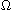be a bounded q-pseudoconvex domain in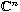,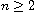and let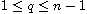. Ifis smooth, we find sufficient conditions for the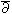-Neumann operator to be compact. Ifis non-smooth and if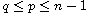, we show that compactness of the-Neumann operator,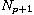, on square integrable (0, p+1)-forms is equivalent to compactness of the commutators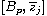,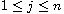, on square integrable-closed (0, p)-forms, where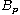is the Bergman projection on (0, p)-forms. Moreover, we prove that compactness of the commutator ofwith bounded functions percolates up in the-complex on-closed forms and square integrable holomorphic forms. Furthermore, we find a characterization of compactness of the canonical solution operator,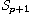, of the-equation restricted on (0, p+1)-forms with holomorphic coefficients in terms of compactness of commutators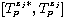,, on (0, p)-forms with holomorphic coefficients, where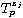is the Bergman-Toeplitz operator acting on (0, p)-forms with symbol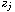. This extends to domains which are not necessarily pseudoconvex.

Submitted August 4, 2017. Published May 10, 2018.
Math Subject Classifications: 32F10, 32W05.
Key Words:and-Neumann operator; Bergman-Toeplitz operator; q-convex domains.

Show me the PDF file (272 KB), TEX file for this article.Sayed Saber Department of Mathematics Faculty of Science and Arts in Baljurashi Albaha University Albaha, Saudi Arabia